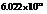# How many moles are there in each sample? a. 55.0 g Ti b. 16.9 mg Na c. 7 .95 × 10 24 Ne atoms d. 25 Xe atoms### Chemistry In Focus

7th Edition
Tro + 1 other
Publisher: Cengage Learning,
ISBN: 9781337399692

#### Solutions

Chapter
Section### Chemistry In Focus

7th Edition
Tro + 1 other
Publisher: Cengage Learning,
ISBN: 9781337399692
Chapter 3, Problem 46E
Textbook Problem
51 views

## How many moles are there in each sample?a. 55.0 g Tib. 16.9 mg Nac. 7 .95  ×  10 24 Ne atomsd. 25 Xe atoms

Interpretation Introduction

Interpretation:

The number of moles present in each sample is to be calculated.

Concept Introduction:

Mole is the unit of the amount of a substance. It relates the number of particles to the molar mass.

One mole of a substance contains Avogadro number (6.022×1023) of particles. The number remains constant regardless of the nature of the substance. One mole of an atom contains 6.022×1023 atoms. One mole of a molecule containsmolecules.

It also relates to the molar mass of substance in grams. The weight of one mole of a substance is equivalent to its molar mass in grams.

To sum up, 1 mole of a substance contains 6.022×1023 particles and weighs the same as its molar mass in grams

### Explanation of Solution

a) 55 g Ti

The calculation for the moles of Ti is as follows:

Molar mass of Ti is 41.88 g.

Number of moles=given massmolar mass

Substitute the values in the above equation:

Hence, the number of moles in Ti is 1.15 mol.

b)16.9 mg Na

Conversion of the mass of Na from mg to g is as follows:

Mass of Na = 16.9 mg1000 mg = 1 g

The number of moles of Na is calculated as follows:

Molar mass of Na is 23 g.

Number of moles=given massmolar mass

Substitute the values in the above equation:

### Still sussing out bartleby?

Check out a sample textbook solution.

See a sample solution

#### The Solution to Your Study Problems

Bartleby provides explanations to thousands of textbook problems written by our experts, many with advanced degrees!

Get Started

Find more solutions based on key concepts
After about 50 years of age, bones begin to lose dens.

Nutrition: Concepts and Controversies - Standalone book (MindTap Course List)

A particle on a spring moves in simple harmonic motion along the x axis between turning points at x1 = 100 cm a...

Physics for Scientists and Engineers, Technology Update (No access codes included)

What percentage of the world ocean is involved in wind-driven surface currents?

Oceanography: An Invitation To Marine Science, Loose-leaf Versin